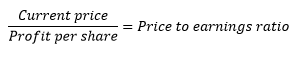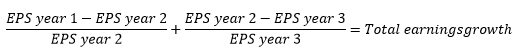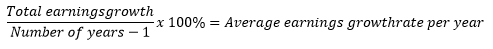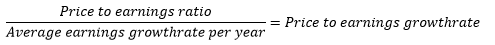# Price/earnings growth

As an investor, you want to make a profit on your investments. The way to do this is to buy the right companies at the right time. And to sell them at the right time. To achieve this main goal, there are a lot of different valuation methods and indicators around. One of these indicators is the profit-to-earnings growth, or PEG, which is based on the price-to-earnings ratio.

## Price/earnings ratio

The result of the price-to-earnings ratio (P/E ratio) is a number. This number tells you how many years it will take with the current profits to repay the current share price. Put differently: how many times can you fit the current earnings-per-share (EPS) in the share price. The calculation of the price-to-earnings ratio is as follows:This price-to-earnings ratio tells you the current valuation of the share. However, this core indicator does not take into account the earnings growth (or decline) of a company. This is why the price-to-earnings growth is used.

## Price/earnings growth

So, to calculate the price-to-earnings growth, you will not only need the latest profit. You will also need to find the growth rate of the earnings per share. Usually, the average growth of the earnings per share over the last years is used. To calculate the growth rate on this site in the companies section, we use the earnings of the last five years.

In the below formulas, we have used the growth rate for the last three years. This is done to limit the complexity of the formulas, but still give you an idea how this works. The average growth of the earnings-per-share (EPS) over the last 3 years, is calculated with the formula below. The ‘Year 1’ is the first your of the last three years.The result of the abovementiond formula gives the price-to-earnings growth indicator. Using the regular price-to-earnings logic, the result is split into three groups: a negative price-to-earnings ratio, a price-to-earnings growth between 0 and 1 and a price-to-earnings growth above 1.

When the price-to-earnings growth is negative, the total growth of the profit over the years used is negative. Differently said, the earnings-per-share have declined. A possible explanation for this is that the company overall earns less. A different explanation is that the number of shares has increased, thus the earnings have to be divided by more shares. This means that when calculating the earnings growth per share, it is not enough to just know the earnings. You should also be aware of the number of shares outstanding. A company that has a negative price-to-earnings growth ratio, is not an interesting investment. Even less so when this decline is expected to continue.

When the price-to-earnings growth ratio is above 1, you can expect that the price of the share is too high. In this case, the price of the share is higher than the earnings-per-share justifies. You can speak of overvaluation. And overvalued shares are usually not a good investment.

When the price-to-earnings growth is between the 0 and 1, you can say the share is undervalued. How closer the price-to-earnings growth is to 0, the more undervalued the share is. The average growth rate per share per year is higher than the price-to-earnings ratio. This results in a share that can be bought based on this indicator.

## Price-to-earnings growth points of attention

You should be aware of a few points of attention when using the price-to-earnings growth indicator. First of all, the price-to-earnings growth indicator is an indicator that looks back several years. To hold firm the earnings per share should continue to grow at the same rate in the years in the future. If an indication exist that the earnings-per-share will decline in the future, you should take this into account while calculating the earnings-per-share growth and your decisions based on this indicator.

A second point of attention is the profit and the number of shares, as already mentioned. The profit per share depends on the profit in a year and the number of outstanding shares. If the profit declines or the number of shares increase, the earnings-per-share will decrease. This means that a company will issue new shares in the near future, you should take this into account when using this indicator for your decisions.

## Compare the price/earnings growth

You can find the price-to-earnings growth indicator of companies listed in The Netherlands in the company tool.

If you want to compare the price-to-earnings growth indicator for different companies, you can use our company compare tool.